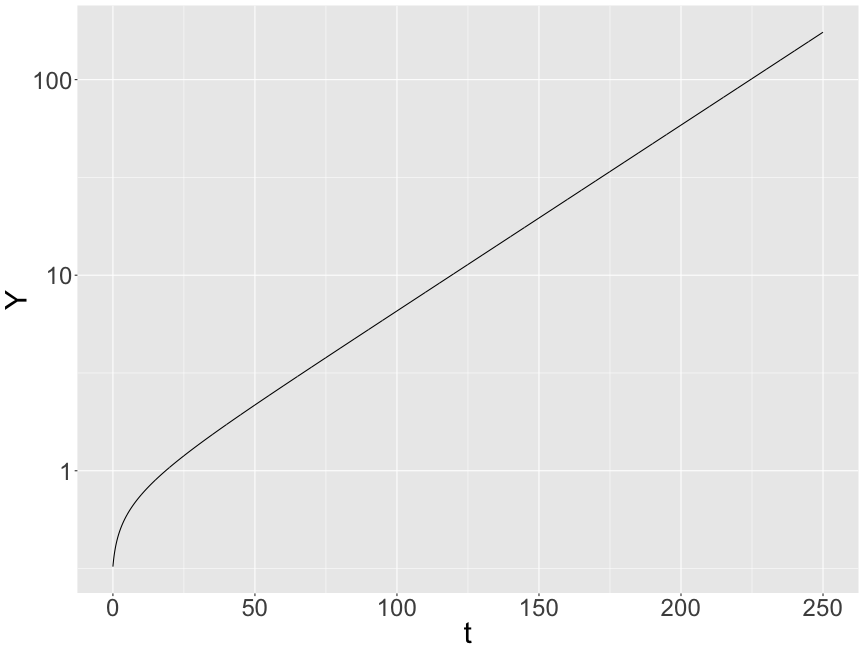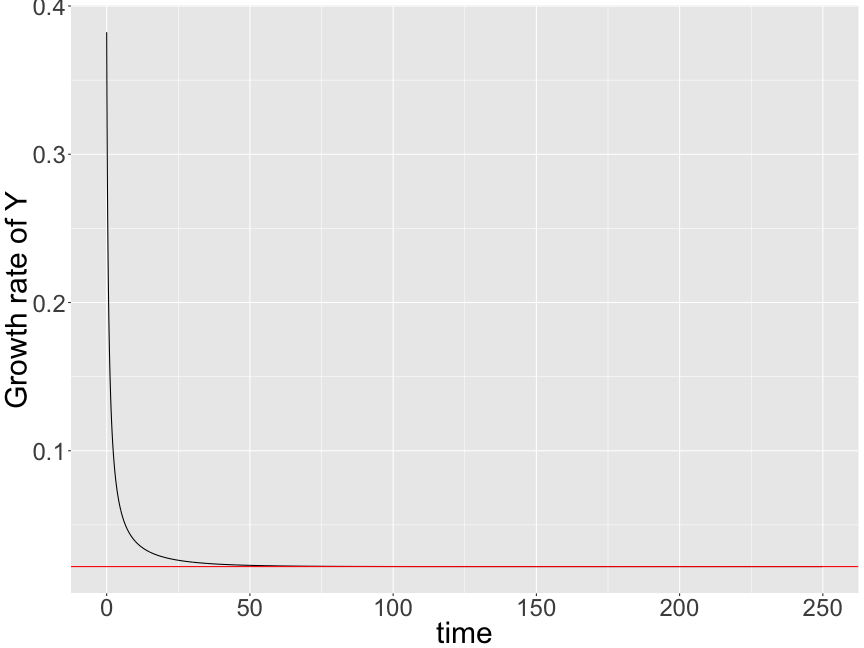# Macroeconomics Q4

Kenji Sato
2016-12-27

### Today's goal

To add decorations to the Solow model.

• Environmental consideration
• Introduction to the Ramsey–Cass-Koopmans Model

### Why incorporate environment in the model of growth?

• The baseline Solow model assumes only two inputs.
• Capital $$K$$
• Effective labor $$AL$$
• Other factors in real production.
• Land
• Natural resources
• Not clear whether sustainable growth is still possible with these limited factors…

### Malthusian economy

• Production with land:
• $$T$$ for amount of land; $$L$$ for labor

$Y = T^\beta L^{(1-\beta)},$

• CRS with all inputs $$(T, L)$$ combined.
• BUT amount of available land is constant (DRS in $$L$$)
• Due to increasing population, $$Y/L$$ tends to zero.

### Natural Resources

• Many natural resources are in finite supply.
• Oil
• Natural gas
• Coal
• Rare metal
• The stock of such nonrenewable resources depletes with the passage of time.

### Solow model and environment

• It is important to estimate the effect of having
such factors as land and nonrenewable resources on economic growth
• If the cost is small, we can be confident that the baseline Solow model is a good model of our economy
• If the cost is large, we know how much effort must be put into environmental prtection.

### Preparation: Another solution method

• Consider the baseline Solow model again.
• Redo the calculation with a different method.
• We will find a path on which all variables have constant growth rates.
• We assume Cobb-Douglas

$F(K, AL) = K^\alpha (AL)^{1 - \alpha}$

### Preparation (cont'd)

Recall that $\dot K = s Y - \delta K$ we have $\frac{\dot K}{K} = s \frac{Y}{K} - \delta$ If $$\dot K / K$$ is constant, $$Y/K$$ must be constant. Thus, $$K$$ and $$Y$$ share the same growth rate.

$g_Y = g_K$

### Preparation (cont'd)

From $Y = K^\alpha (AL)^{1-\alpha}$

we obtain $g_Y = \alpha g_K + (1-\alpha)(g_A + g_L)$ Since $$g_Y = g_K$$, \begin{aligned} g_Y &= g_K = g_A + g_L\\ &= g + n. \end{aligned}

Growth rate for $$Y/L$$ is $$g$$.

• Land $$T$$

$\dot T = 0.$

• Stock of nonrenewable resources is denoted by $$R$$
• $$E$$ the amount of extraction.

$\dot R = - E$

Assume that a constant fraction of the stock of resource is extracted each moment.

$E = b R, \qquad b > 0$

If $$b$$ is large, the resource depletes very quickly.

### Capital Accumulation

As before…

$\dot K = s Y - \delta K.$

If $$Y$$ and $$K$$ grow at constant rates, the growth rates coincide (balanced growth).

### Production function

Assume Cobb-Douglas:

$Y = K^\alpha E^\beta T^\gamma (AL)^{1-\alpha-\beta-\gamma}$

with

\begin{aligned} \alpha, \beta, \gamma &> 0, \\ \alpha + \beta + \gamma &< 1 \end{aligned}

### Growth rates

Let's compute growth rates along the BGP ($$g_Y = g_K$$)

From $Y = K^\alpha E^\beta T^\gamma (AL)^{1-\alpha-\beta-\gamma}$

we have

\begin{aligned} g_Y &= \alpha g_K + \beta g_E + \gamma g_T + (1-\alpha -\beta-\gamma) (g_A + g_L)\\ &= \alpha g_K + \beta g_E + (1-\alpha-\beta-\gamma)(g + n) \end{aligned}

### Growth rates (cont'd)

Noticing that $$g_E = -b$$

$g_Y = \frac{ (1-\alpha-\beta-\gamma)(g+n) - \beta b }{ 1-\alpha }$

Per-capita growth rate:

$g_{Y/L} = g_Y - n = \frac{ (1-\alpha-\beta-\gamma)g - \beta b - (\beta+\gamma)n }{ 1-\alpha }$

### Growth rates (cont'd)

$g_{Y/L} = g_Y - n = \frac{ (1-\alpha-\beta-\gamma)g - \beta b - (\beta+\gamma)n }{ 1-\alpha }$

• The land and resource limitations cause a drag on growth.
• But if technological growth rate $$g$$ is large enough, $$g_{Y/L}$$ can be positive.
• This is what has happened.
• Sustained growth is possible.

### Quantifying the drag

• To quantify the drag due to those limitations, we compare the above-calculated drag with that of a hypothetical economy in which resource and land per worker are constant.
• “[T]o gauge how much these limitations are reducing growth, we need to ask how much greater growth would be if resources and land per worker were constant.” (Romer4e, p. 41)
• Nordhaus (1992)

### Quantifying the drag (cont'd)

• Replace the assumptions on $$T$$ and $$R$$ with:
• $$\dot T = n T$$
• $$\dot R = n R$$

Growth rate of $$Y/L$$ along the BGP (Verify this)

$\tilde g_{Y/L} = \frac{(1-\alpha-\beta-\gamma)g}{1-\alpha}$

The drag is thus
$\tilde g_{Y/L} - g_{Y/L} = \frac{\beta b + (\beta+\gamma)n}{1-\alpha}$

### Quantifying the drag (cont'd)

Calibration according to Nordhaus (1992):

beta = 0.1; gamma = 0.1;
alpha = 0.2; n = 0.01; b = 0.005

drag = (beta * b + (beta + gamma) * n) / (1 - alpha)
drag

 0.003125


The limited land and decreasing nonrenewable resources reduce per-capita growth rate by 0.3 percentage point. A more elaborate model of Nordhaus's estimates smaller drag.

The drag is not trivial but not large.

### Supplement: Stability

We analyzed the BGP for the baseline Solow model because it has global stability. Start from anywhere, you eventually reach that path (in very long run).

As for the environmental Solow model, we bypassed the analysis of transition path and got directly to the BGP. We still don't know whether or not this BGP will is reached in the long run.

Some macroeconomic analyses are based on unstable equilibrium paths …. but that is a bad practice.

I would strongly recommend to check stability somehow. We do this by a computer simulation. It's not rigorous mathematical proof but we can be sure that the above BGP analysis is relevant.

### Simulation

library(dplyr)

s = 0.3;     alpha = 0.2;  beta = 0.1
gamma = 0.1; delta = 0.05; b = 0.005
g = 0.02;    n = 0.01;     dt = 0.05;

t = seq(0.0, 250.0, by=dt)
df = data.frame(t)

df = df %>%
mutate(A = exp(g * t)) %>%
mutate(L = exp(n * t)) %>%
mutate(T = exp(0 * t)) %>%
mutate(E = b * exp(-b * df\$t))

     t        A        L T           E
1 0.00 1.000000 1.000000 1 0.005000000
2 0.05 1.001001 1.000500 1 0.004998750
3 0.10 1.002002 1.001001 1 0.004997501
4 0.15 1.003005 1.001501 1 0.004996251
5 0.20 1.004008 1.002002 1 0.004995002
6 0.25 1.005013 1.002503 1 0.004993754


### Simulation (cont'd)

F = function(K, A, L, T, E){
Y = (K^alpha) * (E^beta) * (T^gamma) * (A*L)^(1-alpha-beta-gamma)
return(Y)
}

K = 0.05
for (i in 1:nrow(df)){
A = df[i, "A"]
L = df[i, "L"]
T = df[i, "T"]
E = df[i, "E"]
Y = F(K, A, L, T, E)

df[i, "K"] = K
df[i, "Y"] = Y
K = K + dt * (s * Y - delta * K)
}

     t        A        L T           E          K         Y
1 0.00 1.000000 1.000000 1 0.005000000 0.05000000 0.3233635
2 0.05 1.001001 1.000500 1 0.004998750 0.05472545 0.3295451
3 0.10 1.002002 1.001001 1 0.004997501 0.05953182 0.3354338
4 0.15 1.003005 1.001501 1 0.004996251 0.06441449 0.3410623
5 0.20 1.004008 1.002002 1 0.004995002 0.06936939 0.3464580
6 0.25 1.005013 1.002503 1 0.004993754 0.07439284 0.3516440


### Simulation (cont'd)

library(ggplot2)
ggplot(data=df, aes(x=t, y=Y)) + geom_line() + scale_y_log10() + theme(text=element_text(size=30))It seems like the equilibrium path is converging to the BGP.

### Simulation (cont'd)

:small-code

growth.rate = (df[2:nrow(df), "Y"] - df[1:(nrow(df)-1), "Y"]) / df[1:(nrow(df)-1), "Y"] / dt
qplot(x=df[1:(nrow(df)-1),"t"], y=growth.rate, geom='line') +
geom_hline(aes(yintercept=((1-alpha-beta-gamma) * (g + n) - beta * b )/ (1 - alpha)), color="red") +
labs(y='Growth rate of Y', x='time') + theme(text=element_text(size=30))### Ramsey-Cass-Koopmans Model

In the Solow model, the saving function is constant.

In the Ramsey-Cass-Koopmans model (or more simply Ramsey model),

• the amount of saving is endogenously determined
• for a certain specification, we can derive the constant saving rate; the Solow model with micro-foundation.

The Ramsey model predicts

• that the growth rate for per-capita income/capital is determined solely by exogenous technology growth rate.
• that is, the prediction of the Solow model is robust.

### Ramsey-Cass-Koopmans Model (cont'd)

There is a subtle difference concerning policy change.

• In the Solow model, a rise of government purchases crowds out investment.
• In the Ramsey model, there is not crowding-out effect.

This difference comes from the fact that the agents in the Ramsey model behave forward-lookingly and take there future income as given.

A permanent policy change alters the total income but doesn't raise substitution between saving and consumption.

### Simple Optimal Growth Model

Before tackling the model in Romer 4e, we analyze the one-sector optimal growth model:

\begin{aligned} \max \int_0^\infty e^{-\rho t} u(c(t)) dt \end{aligned}

subject to

\begin{aligned} \dot k(t) &= f(k(t)) - \delta k(t) - c(t)\\ k(t) &\ge 0\\ c(t) &\ge 0 \end{aligned}

• $$\rho$$: discount rate
• $$u$$: utility function

Maximize discounted sum of utility from consumption stream subject to the capital accumulation constraint

### Simple Optimal Growth Model (cont'd)

We will study

• how to derive this continuous-time optimization from much easier-to-understand discrete-time analog,
• how to solve it analytically (Hamiltonian)
• how to relate it to the model in Romer 4e
• how to solve Ramsey model numerically (Dynamic Programming)